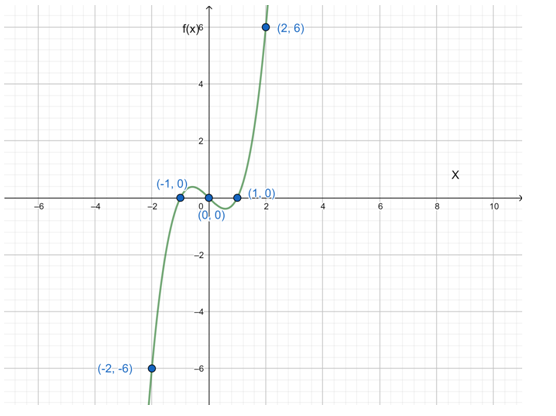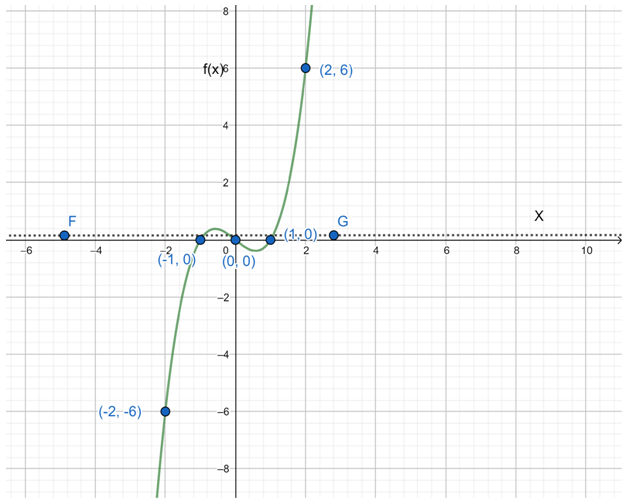# The graph of the function f ( x ) = x 3 − x### Precalculus: Mathematics for Calcu...

6th Edition
Stewart + 5 others
Publisher: Cengage Learning
ISBN: 9780840068071### Precalculus: Mathematics for Calcu...

6th Edition
Stewart + 5 others
Publisher: Cengage Learning
ISBN: 9780840068071

#### Solutions

Chapter 2.7, Problem 65E
To determine

## To sketch: The graph of the function f( x )= x 3 −x

Expert Solution

### Explanation of Solution

Given information f( x )= x 3 x

Graph:

 x 0 -1 2 1 -2 f(x) 0 0 6 0 -6Interpretation:

The horizontal line test is a method that can be used to determine if a function is a one-to-one function. This means that, for every y-value in the function, there is only one unique x-value.

So, here FG line cuts the graph three times.

Thus is not a one-to-one graph.

### Have a homework question?

Subscribe to bartleby learn! Ask subject matter experts 30 homework questions each month. Plus, you’ll have access to millions of step-by-step textbook answers!